Courses

# NEET Previous Year Questions (2014-19): Thermodynamics Notes | EduRev

## NEET : NEET Previous Year Questions (2014-19): Thermodynamics Notes | EduRev

The document NEET Previous Year Questions (2014-19): Thermodynamics Notes | EduRev is a part of the NEET Course Chemistry 28 Years Past year papers for NEET/AIPMT Class 11.
All you need of NEET at this link: NEET

Q.1. Under isothermal condition, a gas at 300 K expands from 0.1 L to 0.25 L against a constant external pressure of 2 bar. The work done by the gas is
(Given that 1 L bar = 100 J)    (2019)
(a) -30 J
(b) 5 kJ
(c) 25 J
(d) 30 J
Ans:
a
∴ Wirr = - Pext ΔV
= - 2 bar × (0.25 – 0.1) L
= - 2 × 0.15
= - 0.30 L bar (1 L bar = 100J)
= - 0.30 × 100 J
= - 30 J

Q.2. In which case change in entropy is negative?    (2019)
(a) Evaporation of water
(b) Expansion of a gas at constant temperature
(c) Sublimation of solid to gas
(d) 2H(g) → H2(g)
Ans
: d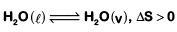• Expansion of gas at constant temperature,  ΔS > 0
• Sublimation of solid to gas, ΔS > 0
• 2H(g) → H2(g), ΔS < 0 (∵ Δng < 0)

Q.3. The bond dissociation energies of X2, Y2 and XY are in the ratio of 1 : 0.5 : 1. ΔH for the formation of XY is -200 kJ mol-1. The bond dissociation energy X2 will be    (2018)
(a) 200 kJ mol-1
(b) 100 kJ mol-1
(c) 800 kJ mol-1

(d) 400 kJ mol-1
Ans:
c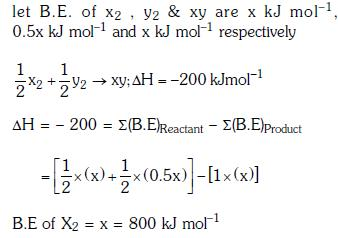Q.4. For a given reaction, ΔH = 35.5 kJ mol-1 and ΔS = 83.6 JK-1mol-1. The reaction is spontaneous at : (Assume that ΔH and ΔS do not vary with tempearature)    (2017)
(a) T > 425 K
(b) T > 298 K
(c) T < 425 K
(d) All temperatures
Ans:
a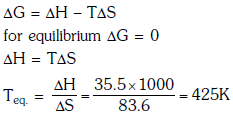Q.5. A gas is allowed to expand in a well insulated container against a constant external pressure of 2.5 atm from an initial volume of 2.50 L to a final volume of 4.50 L. The change in internal energy ΔU of the gas in joules will be:-    (2017)
(a) -500J

(b) -505J
(c) +505J
(d) 1136.25J
Ans:
b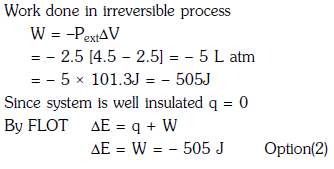Q.6. The correct thermodynamic conditions for the spontaneous reaction at all temperatures is:    (2016)
(a) ΔH < 0 and ΔS < 0
(b) ΔH < 0 and ΔS = 0
(c) ΔH > 0 and ΔS < 0
(d) ΔH < 0 and ΔS > 0
Ans:
d
ΔG = ΔH - TΔS
For spontaneous process (ΔG = -ve) at all temperature, ΔH < 0 & ΔS > 0.

Q.7. Consider the following liquid-vapour equilibrium.
Liquid ⇌ Vapour
Which of the following relations is correct ?    (2016)

(a)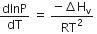(b)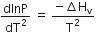(c)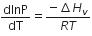(d)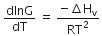Ans:
a
The given phase equilibria is
Liquid ⇌ Vapour
This equilibrium states that, when liquid is heated, it converts into vapour but on cooling, it further converts into liquid, which is derived by Clausius clapeyron and the relationship is written as,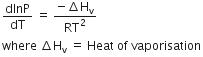Q.8. Which of the following statements is correct for a reversible process in a state of equilibrium ?    (2015)
(a) ΔG
° = 2.30 RT log K
(b) ΔG = -2.30 RT log K
(c) ΔG = 2.30 RT log K
(d) ΔG° = -2.30 RT log K
Ans:
d
ΔG=ΔG° + 2.303 RT log K
0 = ΔG° + 2.303 RT log K
ΔG° = -2.303 RT log K

Q.9. For the reaction: X2O(l) → 2XO2 (g)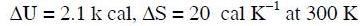Hence, ΔG is :    (2014)
(a) 9.3 k cal
(b) -9.3 k cal
(c) 2.7 k cal
(d) -2.7 k cal
Ans:
d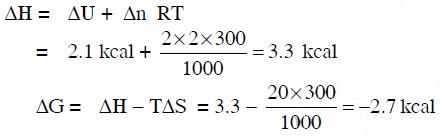Offer running on EduRev: Apply code STAYHOME200 to get INR 200 off on our premium plan EduRev Infinity!

14 docs|25 tests

,

,

,

,

,

,

,

,

,

,

,

,

,

,

,

,

,

,

,

,

,

;